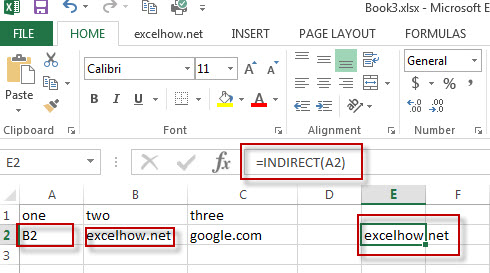# Excel Indirect Function

This post will guide you how to use Excel INDIRECT function with syntax and examples in Microsoft excel.

## Description

The Excel INDIRECT function returns the cell reference based on a text string, such as: type the text string “A2” in B1 cell, it just a text string, so you can use INDIRECT function to convert text string as cell reference.

The INDIRECT function is a build-in function in Microsoft Excel and it is categorized as a Lookup and Reference Function.

The INDIRECT function is available in Excel 2016, Excel 2013, Excel 2010, Excel 2007, Excel 2003, Excel XP, Excel 2000, Excel 2011 for Mac.

## Syntax

The syntax of the INDIRECT function is as below:

`= INDIRECT  (ref_text,[a1])`

Where the INDIRECT function arguments are:
Ref_text -This is a required argument. A reference to a cell as a text string.
A1 – This is an optional argument.  It is a logical value that specify the style of the Ref_text reference.
Note: If A1 value is True or omitted, the ref_text will be interpreted as an A1-style reference.
If A1 value is False, The ref_text will be interpreted as R1C1 style reference.

## Example

The below examples will show you how to use Excel INDIRECT Lookup and Reference Function to return a  cell or range reference that is represented by a text string.

#1 To get the value of the reference in cell A2, just using the following excel formula: =INDIRECT(A2)### More Excel INDIRECT  Function Examples

• Get Cell Value Based on Row and Column Numbers
If you want to retrieve values using cell references based on row and column numbers, you can create a formula based on the INDIRECT function and the ADDRESS function.….
• Sumif with 3D Reference for Multiple Worksheet
If you want to sum 3d references across the different worksheet, and you need to create a complex formula based on the SUMPRODUCT function, the SUMIF function, and the INDIRECT function..….
• Sum Numbers from 1 to N
Assuming that you supply a number 100, and you want to sum the numbers from 1 to 100 (1+2+3+…+100), you need to create a formula based on the SUMPRODUCT function, the ROW function and the INDIRECT function to achieve the result..….